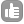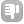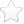0I am currently working on the qNMR concentration of compounds X in commercial samples: The following is my scenario I dissolve 2.67mg of internal standard, MW:116.07g/mol in 1000uL of deuterated solvent, then i pipette out 100uL of the internal standard into another micro centrifuge tube which consist of my compounds X, MW: 332.4g/mol (but unknown which i would like to determine) in commercial samples: I am currently using the following formula Cx=Cm(Ix/Im)(Nm/Nx); C=concentration, Ix=signal intensity of singlet of 2 vinylic protons of maleic acid at 6.18ppm, Im=1(doublet signal of compounds X), what is the molar concentration of my compounds X? I am proceed with the following calculation: n=(2.6710^(-3)/116.07= 2.310^(-5) mol Molarity= [2.310^(-5) mol]/(100010^(-6) L)= 0.023M M1V1=M2V2 0.023M100uL=M2600uL M2=3.8310^(-3)M Cx=3.8310^(-3)M 0.091082=6.98*10^(-4)M 6.9810^(-4)M would have(6.9810^(-4)M*332.4g/mol) 0.232g of compound X in 1L of solvent, therefore in 600uL of solvent, compound X= 139ug, did i do any mistake in my concentration calculation??? But the problem is i tested this compound in three analytical instrument, which are HPLC and LCMS. All of this three instrument all of them giving me different amount, so I do not know which instrument to rely on. asked Sep 24 '16 at 19:060Hello Kong Ka Ling, qNMR is sometimes a bit tricky! For quantification you must be aware of the relaxation and other stuff, ... causing different values ... more information about qNMR: http://nmr-analysis.blogspot.de/2009/11/basis-on-qnmr-integration-rudiments.html http://u-of-o-nmr-facility.blogspot.de/search/label/quantitative%201H%20NMR http://mestrelab.com/blog/what-is-qnmr/ http://gfp.people.uic.edu/qnmr/ MestreNOVA has got a special plugin, ... you can use the 31P-Sensitivity Standard (0.0485 M TPP in Acetone-D6) ... correct relaxation rate and then you can calculate a machine/spectrometer correction factor. So you can measure with this factor the molarities, ... Yours sincerely, Ulrich answered Oct 13 '16 at 06:38Ulrich Haunz 210

 toggle preview

Tags:

×4
×2

Asked: Sep 24 '16 at 19:06

Seen: 12,311 times

Last updated: Oct 13 '16 at 06:38